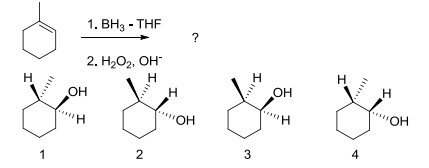# Problem: Which of the following would be produced from the following reaction? a) 1 and 4 b) 1, 2, 3, and 4 c) 3 and 4 d) 2 and 3 e) 1 and 2

###### FREE Expert Solution
100% (54 ratings)
###### Problem Details

Which of the following would be produced from the following reaction?

a) 1 and 4

b) 1, 2, 3, and 4

c) 3 and 4

d) 2 and 3

e) 1 and 2Frequently Asked Questions

What scientific concept do you need to know in order to solve this problem?

Our tutors have indicated that to solve this problem you will need to apply the Hydroboration concept. You can view video lessons to learn Hydroboration. Or if you need more Hydroboration practice, you can also practice Hydroboration practice problems.

What is the difficulty of this problem?

Our tutors rated the difficulty ofWhich of the following would be produced from the following ...as high difficulty.

How long does this problem take to solve?

Our expert Organic tutor, Chris took 2 minutes and 21 seconds to solve this problem. You can follow their steps in the video explanation above.

What professor is this problem relevant for?

Based on our data, we think this problem is relevant for Professor Kell's class at UIUC.